# Tag: Galois

In The Da Vinci Code, Dan Brown feels he need to bring in a French cryptologist, Sophie Neveu, to explain the mystery behind this series of numbers:

13 – 3 – 2 – 21 – 1 – 1 – 8 – 5

The Fibonacci sequence, 1-1-2-3-5-8-13-21-34-55-89-144-… is such that any number in it is the sum of the two previous numbers.

It is the most famous of all integral linear recursive sequences, that is, a sequence of integers

$a = (a_0,a_1,a_2,a_3,\dots)$

such that there is a monic polynomial with integral coefficients of a certain degree $n$

$f(x) = x^n + b_1 x^{n-1} + b_2 x^{n-2} + \dots + b_{n-1} x + b_n$

such that for every integer $m$ we have that

$a_{m+n} + b_1 a_{m+n-1} + b_2 a_{m+n-2} + \dots + b_{n-1} a_{m+1} + a_m = 0$

For the Fibonacci series $F=(F_0,F_1,F_2,\dots)$, this polynomial can be taken to be $x^2-x-1$ because
$F_{m+2} = F_{m+1}+F_m$

The set of all integral linear recursive sequences, let’s call it $\Re(\mathbb{Z})$, is a beautiful object of great complexity.

For starters, it is a ring. That is, we can add and multiply such sequences. If

$a=(a_0,a_1,a_2,\dots),~\quad \text{and}~\quad a’=(a’_0,a’_1,a’_2,\dots)~\quad \in \Re(\mathbb{Z})$

then the sequences

$a+a’ = (a_0+a’_0,a_1+a’_1,a_2+a’_2,\dots) \quad \text{and} \quad a \times a’ = (a_0.a’_0,a_1.a’_1,a_2.a’_2,\dots)$

are again linear recursive. The zero and unit in this ring are the constant sequences $0=(0,0,\dots)$ and $1=(1,1,\dots)$.

So far, nothing terribly difficult or exciting.

It follows that $\Re(\mathbb{Z})$ has a co-unit, that is, a ring morphism

$\epsilon~:~\Re(\mathbb{Z}) \rightarrow \mathbb{Z}$

sending a sequence $a = (a_0,a_1,\dots)$ to its first entry $a_0$.

It’s a bit more difficult to see that $\Re(\mathbb{Z})$ also has a co-multiplication

$\Delta~:~\Re(\mathbb{Z}) \rightarrow \Re(\mathbb{Z}) \otimes_{\mathbb{Z}} \Re(\mathbb{Z})$
with properties dual to those of usual multiplication.

To describe this co-multiplication in general will have to await another post. For now, we will describe it on the easier ring $\Re(\mathbb{Q})$ of all rational linear recursive sequences.

For such a sequence $q = (q_0,q_1,q_2,\dots) \in \Re(\mathbb{Q})$ we consider its Hankel matrix. From the sequence $q$ we can form symmetric $k \times k$ matrices such that the opposite $i+1$-th diagonal consists of entries all equal to $q_i$
$H_k(q) = \begin{bmatrix} q_0 & q_1 & q_2 & \dots & q_{k-1} \\ q_1 & q_2 & & & q_k \\ q_2 & & & & q_{k+1} \\ \vdots & & & & \vdots \\ q_{k-1} & q_k & q_{k+1} & \dots & q_{2k-2} \end{bmatrix}$
The Hankel matrix of $q$, $H(q)$ is $H_k(q)$ where $k$ is maximal such that $det~H_k(q) \not= 0$, that is, $H_k(q) \in GL_k(\mathbb{Q})$.

Let $S(q)=(s_{ij})$ be the inverse of $H(q)$, then the co-multiplication map
$\Delta~:~\Re(\mathbb{Q}) \rightarrow \Re(\mathbb{Q}) \otimes \Re(\mathbb{Q})$
sends the sequence $q = (q_0,q_1,\dots)$ to
$\Delta(q) = \sum_{i,j=0}^{k-1} s_{ij} (D^i q) \otimes (D^j q)$
where $D$ is the shift operator on sequence
$D(a_0,a_1,a_2,\dots) = (a_1,a_2,\dots)$

If $a \in \Re(\mathbb{Z})$ is such that $H(a) \in GL_k(\mathbb{Z})$ then the same formula gives $\Delta(a)$ in $\Re(\mathbb{Z})$.

For the Fibonacci sequences $F$ the Hankel matrix is
$H(F) = \begin{bmatrix} 1 & 1 \\ 1& 2 \end{bmatrix} \in GL_2(\mathbb{Z}) \quad \text{with inverse} \quad S(F) = \begin{bmatrix} 2 & -1 \\ -1 & 1 \end{bmatrix}$
and therefore
$\Delta(F) = 2 F \otimes ~F – DF \otimes F – F \otimes DF + DF \otimes DF$
There’s a lot of number theoretic and Galois-information encoded into the co-multiplication on $\Re(\mathbb{Q})$.

To see this we will describe the co-multiplication on $\Re(\overline{\mathbb{Q}})$ where $\overline{\mathbb{Q}}$ is the field of all algebraic numbers. One can show that

$\Re(\overline{\mathbb{Q}}) \simeq (\overline{\mathbb{Q}}[ \overline{\mathbb{Q}}_{\times}^{\ast}] \otimes \overline{\mathbb{Q}}[d]) \oplus \sum_{i=0}^{\infty} \overline{\mathbb{Q}} S_i$

Here, $\overline{\mathbb{Q}}[ \overline{\mathbb{Q}}_{\times}^{\ast}]$ is the group-algebra of the multiplicative group of non-zero elements $x \in \overline{\mathbb{Q}}^{\ast}_{\times}$ and each $x$, which corresponds to the geometric sequence $x=(1,x,x^2,x^3,\dots)$, is a group-like element
$\Delta(x) = x \otimes x \quad \text{and} \quad \epsilon(x) = 1$

$\overline{\mathbb{Q}}[d]$ is the universal Lie algebra of the $1$-dimensional Lie algebra on the primitive element $d = (0,1,2,3,\dots)$, that is
$\Delta(d) = d \otimes 1 + 1 \otimes d \quad \text{and} \quad \epsilon(d) = 0$

Finally, the co-algebra maps on the elements $S_i$ are given by
$\Delta(S_i) = \sum_{j=0}^i S_j \otimes S_{i-j} \quad \text{and} \quad \epsilon(S_i) = \delta_{0i}$

That is, the co-multiplication on $\Re(\overline{\mathbb{Q}})$ is completely known. To deduce from it the co-multiplication on $\Re(\mathbb{Q})$ we have to consider the invariants under the action of the absolute Galois group $Gal(\overline{\mathbb{Q}}/\mathbb{Q})$ as
$\Re(\overline{\mathbb{Q}})^{Gal(\overline{\mathbb{Q}}/\mathbb{Q})} \simeq \Re(\mathbb{Q})$

Unlike the Fibonacci sequence, not every integral linear recursive sequence has an Hankel matrix with determinant $\pm 1$, so to determine the co-multiplication on $\Re(\mathbb{Z})$ is even a lot harder, as we will see another time.

Reference: Richard G. Larson, Earl J. Taft, ‘The algebraic structure of linearly recursive sequences under Hadamard product’

Sunday january 2nd around 18hr NeB-stats went crazy.

Referrals clarified that the post ‘What is the knot associated to a prime?’ was picked up at Reddit/math and remained nr.1 for about a day.

Now, the dust has settled, so let’s learn from the experience.A Reddit-mention is to a blog what doping is to a sporter.

You get an immediate boost in the most competitive of all blog-stats, the number of unique vistors (blue graph), but is doesn’t result in a long-term effect, and, it may even be harmful to more essential blog-stats, such as the average time visitors spend on your site (yellow graph).

For NeB the unique vistors/day fluctuate normally around 300, but peaked to 1295 and 1733 on the ‘Reddit-days’. In contrast, the avg. time on site is normally around 3 minutes, but dropped the same days to 44 and 30 seconds!

Whereas some of the Reddits spend enough time to read the post and comment on it, the vast majority zap from one link to the next. Having monitored the Reddit/math page for two weeks, I’m convinced that post only made it because it was visually pretty good. The average Reddit/math-er is a viewer more than a reader…

So, should I go for shorter, snappier, more visual posts?

Let’s compare Reddits to those coming from the three sites giving NeB most referrals : Google search, MathOverflow and Wikipedia.This is the traffic coming from Reddit/math, as always the blue graph are the unique visitors, the yellow graph their average time on site, blue-scales to the left, yellow-scales to the right.

Here’s the same graph for Google search. The unique visitors/day fluctuate around 50 and their average time on site about 2 minutes.The math-related search terms most used were this month : ‘functor of point approach’, ‘profinite integers’ and ‘bost-connes sytem’.

More rewarding to me are referrals from MathOverflow.The number of visitors depends on whether the MathO-questions made it to the front-page (for example, the 80 visits on december 15, came from the What are dessins d’enfants?-topic getting an extra comment that very day, and having two references to NeB-posts : The best rejected proposal ever and Klein’s dessins d’enfant and the buckyball), but even older MathO-topics give a few referrals a day, and these people sure take their time reading the posts (+ 5 minutes).

Other MathO-topics giving referrals this month were Most intricate and most beautiful structures in mathematics (linking to Looking for F-un), What should be learned in a first serious schemes course? (linking to Mumford’s treasure map (btw. one of the most visited NeB-posts ever)), How much of scheme theory can you visualize? (linking again to Mumford’s treasure map) and Approaches to Riemann hypothesis using methods outside number theory (linking to the Bost-Connes series).

Finally, there’s Wikipediagiving 5 to 10 referrals a day, with a pretty good time-on-site average (around 4 minutes, peaking to 12 minutes). It is rewarding to see NeB-posts referred to in as diverse Wikipedia-topics as ‘Fifteen puzzle’, ‘Field with one element’, ‘Evariste Galois’, ‘ADE classification’, ‘Monster group’, ‘Arithmetic topology’, ‘Dessin d’enfant’, ‘Groupoid’, ‘Belyi’s theorem’, ‘Modular group’, ‘Cubic surface’, ‘Esquisse d’un programme’, ‘N-puzzle’, ‘Shabat polynomial’ and ‘Mathieu group’.

What lesson should be learned from all this data? Should I go for shorter, snappier and more visual posts, or should I focus on the small group of visitors taking their time reading through a longer post, and don’t care about the appallingly high bounce rate the others cause?

This is a belated response to a Math-Overflow exchange between Thomas Riepe and Chandan Singh Dalawat asking for a possible connection between Connes’ noncommutative geometry approach to the Riemann hypothesis and the Langlands program.

Here’s the punchline : a large chunk of the Connes-Marcolli book Noncommutative Geometry, Quantum Fields and Motives can be read as an exploration of the noncommutative boundary to the Langlands program (at least for $GL_1$ and $GL_2$ over the rationals $\mathbb{Q}$).

Recall that Langlands for $GL_1$ over the rationals is the correspondence, given by the Artin reciprocity law, between on the one hand the abelianized absolute Galois group

$Gal(\overline{\mathbb{Q}}/\mathbb{Q})^{ab} = Gal(\mathbb{Q}(\mu_{\infty})/\mathbb{Q}) \simeq \hat{\mathbb{Z}}^*$

and on the other hand the connected components of the idele classes

$\mathbb{A}^{\ast}_{\mathbb{Q}}/\mathbb{Q}^{\ast} = \mathbb{R}^{\ast}_{+} \times \hat{\mathbb{Z}}^{\ast}$

The locally compact Abelian group of idele classes can be viewed as the nice locus of the horrible quotient space of adele classes $\mathbb{A}_{\mathbb{Q}}/\mathbb{Q}^{\ast}$. There is a well-defined map

$\mathbb{A}_{\mathbb{Q}}’/\mathbb{Q}^{\ast} \rightarrow \mathbb{R}_{+} \qquad (x_{\infty},x_2,x_3,\ldots) \mapsto | x_{\infty} | \prod | x_p |_p$

from the subset $\mathbb{A}_{\mathbb{Q}}’$ consisting of adeles of which almost all terms belong to $\mathbb{Z}_p^{\ast}$. The inverse image of this map over $\mathbb{R}_+^{\ast}$ are precisely the idele classes $\mathbb{A}^{\ast}_{\mathbb{Q}}/\mathbb{Q}^{\ast}$. In this way one can view the adele classes as a closure, or ‘compactification’, of the idele classes.

This is somewhat reminiscent of extending the nice action of the modular group on the upper-half plane to its badly behaved action on the boundary as in the Manin-Marcolli cave post.

The topological properties of the fiber over zero, and indeed of the total space of adele classes, are horrible in the sense that the discrete group $\mathbb{Q}^*$ acts ergodically on it, due to the irrationality of $log(p_1)/log(p_2)$ for primes $p_i$. All this is explained well (in the semi-local case, that is using $\mathbb{A}_Q’$ above) in the Connes-Marcolli book (section 2.7).

In much the same spirit as non-free actions of reductive groups on algebraic varieties are best handled using stacks, such ergodic actions are best handled by the tools of noncommutative geometry. That is, one tries to get at the geometry of $\mathbb{A}_{\mathbb{Q}}/\mathbb{Q}^{\ast}$ by studying an associated non-commutative algebra, the skew-ring extension of the group-ring of the adeles by the action of $\mathbb{Q}^*$ on it. This algebra is known to be Morita equivalent to the Bost-Connes algebra which is the algebra featuring in Connes’ approach to the Riemann hypothesis.

It shouldn’t thus come as a major surprise that one is able to recover the other side of the Langlands correspondence, that is the Galois group $Gal(\mathbb{Q}(\mu_{\infty})/\mathbb{Q})$, from the Bost-Connes algebra as the symmetries of certain states.

In a similar vein one can read the Connes-Marcolli $GL_2$-system (section 3.7 of their book) as an exploration of the noncommutative closure of the Langlands-space $GL_2(\mathbb{A}_{\mathbb{Q}})/GL_2(\mathbb{Q})$.

At the moment I’m running a master-seminar noncommutative geometry trying to explain this connection in detail. But, we’re still in the early phases, struggling with the topology of ideles and adeles, reciprocity laws, L-functions and the lot. Still, if someone is interested I might attempt to post some lecture notes here.

The Knight-seating problems asks for a consistent placing of n-th Knight at an odd root of unity, compatible with the two different realizations of the algebraic closure of the field with two elements.
The first identifies the multiplicative group of its non-zero elements with the group of all odd complex roots of unity, under complex multiplication. The second uses Conway’s ‘simplicity rules’ to define an addition and multiplication on the set of all ordinal numbers.

The odd Knights of the round table-problem asks for a specific one-to-one correspondence between two realizations of ‘the’ algebraic closure $\overline{\mathbb{F}_2}$ of the field of two elements.

The first identifies the multiplicative group of its non-zero elements with the group of all odd complex roots of unity, under complex multiplication. The addition on $\overline{\mathbb{F}_2}$ is then recovered by inducing an involution on the odd roots, pairing the one corresponding to x to the one corresponding to x+1.

The second uses Conway’s ‘simplicity rules’ to define an addition and multiplication on the set of all ordinal numbers. Conway proves in ONAG that this becomes an algebraically closed field of characteristic two and that $\overline{\mathbb{F}_2}$ is the subfield of all ordinals smaller than $\omega^{\omega^{\omega}}$. The finite ordinals (the natural numbers) form the quadratic closure of $\mathbb{F}_2$.

On the natural numbers the Conway-addition is binary addition without carrying and Conway-multiplication is defined by the properties that two different Fermat-powers $N=2^{2^i}$ multiply as they do in the natural numbers, and, Fermat-powers square to its sesquimultiple, that is $N^2=\frac{3}{2}N$. Moreover, all natural numbers smaller than $N=2^{2^{i}}$ form a finite field $\mathbb{F}_{2^{2^i}}$. Using distributivity, one can write down a multiplication table for all 2-powers.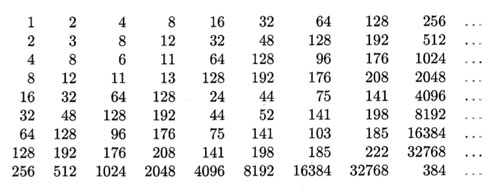The Knight-seating problems asks for a consistent placing of n-th Knight $K_n$ at an odd root of unity, compatible with the two different realizations of $\overline{\mathbb{F}_2}$. Last time, we were able to place the first 15 Knights as below, and asked where you would seat $K_{16}$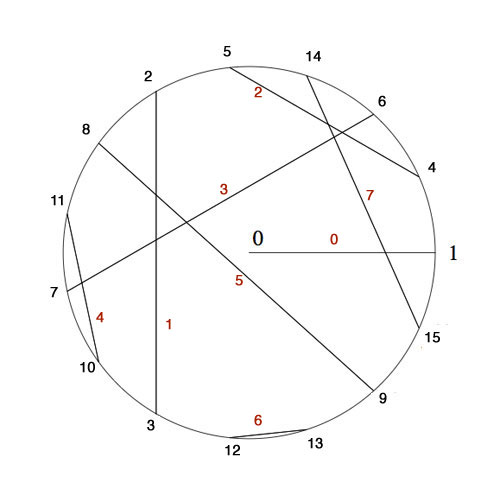$K_4$ was placed at $e^{2\pi i/15}$ as 4 was the smallest number generating the ‘Fermat’-field $\mathbb{F}_{2^{2^2}}$ (with multiplicative group of order 15) subject to the compatibility relation with the generator 2 of the smaller Fermat-field $\mathbb{F}_2$ (with group of order 15) that $4^5=2$.

To include the next Fermat-field $\mathbb{F}_{2^{2^3}}$ (with multiplicative group of order 255) consistently, we need to find the smallest number n generating the multiplicative group and satisfying the compatibility condition $n^{17}=4$. Let’s first concentrate on finding the smallest generator : as 2 is a generator for 1st Fermat-field $\mathbb{F}_{2^{2^1}}$ and 4 a generator for the 2-nd Fermat-field $\mathbb{F}_{2^{2^2}}$ a natural conjecture might be that 16 is a generator for the 3-rd Fermat-field $\mathbb{F}_{2^{2^3}}$ and, more generally, that $2^{2^i}$ would be a generator for the next field $\mathbb{F}_{2^{2^{i+1}}}$.

However, an “exercise” in the 1978-paper by Hendrik Lenstra Nim multiplication asks : “Prove that $2^{2^i}$ is a primitive root in the field $\mathbb{F}_{2^{2^{i+1}}}$ if and only if i=0 or 1.”

I’ve struggled with several of the ‘exercises’ in Lenstra’s paper to the extend I feared Alzheimer was setting in, only to find out, after taking pen and paper and spending a considerable amount of time calculating, that they are indeed merely exercises, when looked at properly… (Spoiler-warning : stop reading now if you want to go through this exercise yourself).

In the picture above I’ve added in red the number $x(x+1)=x^2+1$ to each of the involutions. Clearly, for each pair these numbers are all distinct and we see that for the indicated pairing they make up all numbers strictly less than 8.

By Conway’s simplicity rules (or by checking) the pair (16,17) gives the number 8. In other words, the equation
$x^2+x+8$ is an irreducible polynomial over $\mathbb{F}_{16}$ having as its roots in $\mathbb{F}_{256}$ the numbers 16 and 17. But then, 16 and 17 are conjugated under the Galois-involution (the Frobenius $y \mapsto y^{16}$). That is, we have $16^{16}=17$ and $17^{16}=16$ and hence $16^{17}=8$. Now, use the multiplication table in $\mathbb{F}_{16}$ given in the previous post (or compute!) to see that 8 is of order 5 (and NOT a generator). As a consequence, the multiplicative order of 16 is 5×17=85 and so 16 cannot be a generator in $\mathbb{F}_{256}$.
For general i one uses the fact that $2^{2^i}$ and $2^{2^i}+1$ are the roots of the polynomial $x^2+x+\prod_{j<i} 2^{2^j}$ over $\mathbb{F}_{2^{2^i}}$ and argues as before.

Right, but then what is the minimal generator satisfying $n^{17}=4$? By computing we see that the pairings of all numbers in the range 16…31 give us all numbers in the range 8…15 and by the above argument this implies that the 17-th powers of all numbers smaller than 32 must be different from 4. But then, the smallest candidate is 32 and one verifies that indeed $32^{17}=4$ (use the multiplication table given before).

Hence, we must place Knight $K_{32}$ at root $e^{2 \pi i/255}$ and place the other Knights prior to the 256-th at the corresponding power of 32. I forgot the argument I used to find-by-hand the requested place for Knight 16, but one can verify that $32^{171}=16$ so we seat $K_{16}$ at root $e^{342 \pi i/255}$.

But what about Knight $K_{256}$? Well, by this time I was quite good at squaring and binary representations of integers, but also rather tired, and decided to leave that task to the computer.

If we denote Nim-addition and multiplication by $\oplus$ and $\otimes$, then Conway’s simplicity results in ONAG establish a field-isomorphism between $~(\mathbb{N},\oplus,\otimes)$ and the field $\mathbb{F}_2(x_0,x_1,x_2,\ldots )$ where the $x_i$ satisfy the Artin-Schreier equations

$x_i^2+x_i+\prod_{j < i} x_j = 0$

and the i-th Fermat-field $\mathbb{F}_{2^{2^i}}$ corresponds to $\mathbb{F}_2(x_0,x_1,\ldots,x_{i-1})$. The correspondence between numbers and elements from these fields is given by taking $x_i \mapsto 2^{2^i}$. But then, wecan write every 2-power as a product of the $x_i$ and use the binary representation of numbers to perform all Nim-calculations with numbers in these fields.

Therefore, a quick and dirty way (and by no means the most efficient) to do Nim-calculations in the next Fermat-field consisting of all numbers smaller than 65536, is to use sage and set up the field $\mathbb{F}_2(x_0,x_1,x_2,x_3)$ by

R.< x,y,z,t > =GF(2)[]
S.< a,b,c,d >=R.quotient((x^2+x+1,y^2+y+x,z^2+z+x*y,t^2+t+x*y*z))


To find the smallest number generating the multiplicative group and satisfying the additional compatibility condition $n^{257}=32$ we have to find the smallest binary number $i_1i_2 \ldots i_{16}$ (larger than 255) satisfying

(i1*a*b*c*t+i2*b*c*t+i3*a*c*t+i4*c*t+i5*a*b*t+i6*b*t+
i7*a*t+i8*t+i9*a*b*c+i10*b*c+i11*a*c+i12*c+i13*a*b+
i14*b+i15*a+i16)^257=a*c


It takes a 2.4GHz 2Gb-RAM MacBook not that long to decide that the requested generator is 1051 (killing another optimistic conjecture that these generators might be 2-powers). So, we seat Knight
$K_{1051}$ at root $e^{2 \pi i/65535}$ and can then arrange seatings for all Knight queued up until we reach the 65536-th! In particular, the first Knight we couldn’t place before, that is Knight $K_{256}$, will be seated at root $e^{65826 \pi i/65535}$.

If you’re lucky enough to own a computer with more RAM, or have the patience to make the search more efficient and get the seating arrangement for the next Fermat-field, please drop a comment.

I’ll leave you with another Lenstra-exercise which shouldn’t be too difficult for you to solve now : “Prove that $x^3=2^{2^i}$ has three solutions in $\mathbb{N}$ for each $i \geq 2$.”

Next time you visit your math-library, please have a look whether these books are still on the shelves : Michiel Hazewinkel‘s Formal groups and applications, William Fulton’s and Serge Lange’s Riemann-Roch algebra and Donald Knutson’s lambda-rings and the representation theory of the symmetric group.

I wouldn’t be surprised if one or more of these books are borrowed out, probably all of them to the same person. I’m afraid I’m that person in Antwerp…

Lately, there’s been a renewed interest in $\lambda$-rings and the endo-functor W assigning to a commutative algebra its ring of big Witt vectors, following Borger’s new proposal for a geometry over the absolute point.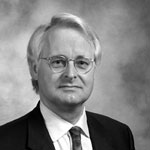However, as Hendrik Lenstra writes in his 2002 course-notes on the subject Construction of the ring of Witt vectors : “The literature on the functor W is in a somewhat unsatisfactory state: nobody seems to have any interest in Witt vectors beyond applying them for a purpose, and they are often treated in appendices to papers devoting to something else; also, the construction usually depends on a set of implicit or unintelligible formulae. Apparently, anybody who wishes to understand Witt vectors needs to construct them personally. That is what is now happening to myself.”

Before doing a series on Borger’s paper, we’d better run through Lenstra’s elegant construction in a couple of posts. Let A be a commutative ring and consider the multiplicative group of all ‘one-power series’ over it $\Lambda(A)=1+t A[[t]]$. Our aim is to define a commutative ring structure on $\Lambda(A)$ taking as its ADDITION the MULTIPLICATION of power series.

That is, if $u(t),v(t) \in \Lambda(A)$, then we define our addition $u(t) \oplus v(t) = u(t) \times v(t)$. This may be slightly confusing as the ZERO-element in $\Lambda(A),\oplus$ will then turn be the constant power series 1…

We are now going to define a multiplication $\otimes$ on $\Lambda(A)$ which is distributively with respect to $\oplus$ and turns $\Lambda(A)$ into a commutative ring with ONE-element the series $~(1-t)^{-1}=1+t+t^2+t^3+\ldots$.

We will do this inductively, so consider $\Lambda_n(A)$ the (classes of) one-power series truncated at term n, that is, the kernel of the natural augmentation map between the multiplicative group-units $~A[t]/(t^{n+1})^* \rightarrow A^*$.
Again, taking multiplication in $A[t]/(t^{n+1})$ as a new addition rule $\oplus$, we see that $~(\Lambda_n(A),\oplus)$ is an Abelian group, whence a $\mathbb{Z}$-module.

For all elements $a \in A$ we have a scaling operator $\phi_a$ (sending $t \rightarrow at$) which is an A-ring endomorphism of $A[t]/(t^{n+1})$, in particular multiplicative wrt. $\times$. But then, $\phi_a$ is an additive endomorphism of $~(\Lambda_n(A),\oplus)$, so is an element of the endomorphism-RING $End_{\mathbb{Z}}(\Lambda_n(A))$. Because composition (being the multiplication in this endomorphism ring) of scaling operators is clearly commutative ($\phi_a \circ \phi_b = \phi_{ab}$) we can define a commutative RING $E$ being the subring of $End_{\mathbb{Z}}(\Lambda_n(A))$ generated by the operators $\phi_a$.

The action turns $~(\Lambda_n(A),\oplus)$ into an E-module and we define an E-module morphism $E \rightarrow \Lambda_n(A)$ by $\phi_a \mapsto \phi_a((1-t)^{-1}) = (1-at)^{-a}$.

All of this looks pretty harmless, but the upshot is that we have now equipped the image of this E-module morphism, say $L_n(A)$ (which is the additive subgroup of $~(\Lambda_n(A),\oplus)$ generated by the elements $~(1-at)^{-1}$) with a commutative multiplication $\otimes$ induced by the rule $~(1-at)^{-1} \otimes (1-bt)^{-1} = (1-abt)^{-1}$.

Explicitly, $L_n(A)$ is the set of one-truncated polynomials $u(t)$ with coefficients in $A$ such that one can find elements $a_1,\ldots,a_k \in A$ such that $u(t) \equiv (1-a_1t)^{-1} \times \ldots \times (1-a_k)^{-1}~mod~t^{n+1}$. We multiply $u(t)$ with another such truncated one-polynomial $v(t)$ (taking elements $b_1,b_2,\ldots,b_l \in A$) via

$u(t) \otimes v(t) = ((1-a_1t)^{-1} \oplus \ldots \oplus (1-a_k)^{-1}) \otimes ((1-b_1t)^{-1} \oplus \ldots \oplus (1-b_l)^{-1})$

and using distributivity and the multiplication rule this gives the element $\prod_{i,j} (1-a_ib_jt)^{-1}~mod~t^{n+1} \in L_n(A)$.
Being a ring-qutient of $E$ we have that $~(L_n(A),\oplus,\otimes)$ is a commutative ring, and, from the construction it is clear that $L_n$ behaves functorially.

For rings $A$ such that $L_n(A)=\Lambda_n(A)$ we are done, but in general $L_n(A)$ may be strictly smaller. The idea is to use functoriality and do the relevant calculations in a larger ring $A \subset B$ where we can multiply the two truncated one-polynomials and observe that the resulting truncated polynomial still has all its coefficients in $A$.

Here’s how we would do this over $\mathbb{Z}$ : take two irreducible one-polynomials u(t) and v(t) of degrees r resp. s smaller or equal to n. Then over the complex numbers we have
$u(t)=(1-\alpha_1t) \ldots (1-\alpha_rt)$ and $v(t)=(1-\beta_1) \ldots (1-\beta_st)$. Then, over the field $K=\mathbb{Q}(\alpha_1,\ldots,\alpha_r,\beta_1,\ldots,\beta_s)$ we have that $u(t),v(t) \in L_n(K)$ and hence we can compute their product $u(t) \otimes v(t)$ as before to be $\prod_{i,j}(1-\alpha_i\beta_jt)^{-1}~mod~t^{n+1}$. But then, all coefficients of this truncated K-polynomial are invariant under all permutations of the roots $\alpha_i$ and the roots $\beta_j$ and so is invariant under all elements of the Galois group. But then, these coefficients are algebraic numbers in $\mathbb{Q}$ whence integers. That is, $u(t) \otimes v(t) \in \Lambda_n(\mathbb{Z})$. It should already be clear from this that the rings $\Lambda_n(\mathbb{Z})$ contain a lot of arithmetic information!

For a general commutative ring $A$ we will copy this argument by considering a free overring $A^{(\infty)}$ (with 1 as one of the base elements) by formally adjoining roots. At level 1, consider $M_0$ to be the set of all non-constant one-polynomials over $A$ and consider the ring

$A^{(1)} = \bigotimes_{f \in M_0} A[X]/(f) = A[X_f, f \in M_0]/(f(X_f) , f \in M_0)$

The idea being that every one-polynomial $f \in M_0$ now has one root, namely $\alpha_f = \overline{X_f}$ in $A^{(1)}$. Further, $A^{(1)}$ is a free A-module with basis elements all $\alpha_f^i$ with $0 \leq i < deg(f)$.

Good! We now have at least one root, but we can continue this process. At level 2, $M_1$ will be the set of all non-constant one-polynomials over $A^{(1)}$ and we use them to construct the free overring $A^{(2)}$ (which now has the property that every $f \in M_0$ has at least two roots in $A^{(2)}$). And, again, we repeat this process and obtain in succession the rings $A^{(3)},A^{(4)},\ldots$. Finally, we define $A^{(\infty)} = \underset{\rightarrow}{lim}~A^{(i)}$ having the property that every one-polynomial over A splits entirely in linear factors over $A^{(\infty)}$.

But then, for all $u(t),v(t) \in \Lambda_n(A)$ we can compute $u(t) \otimes v(t) \in \Lambda_n(A^{(\infty)})$. Remains to show that the resulting truncated one-polynomial has all its entries in A. The ring $A^{(\infty)} \otimes_A A^{(\infty)}$ contains two copies of $A^{(\infty)}$ namely $A^{(\infty)} \otimes 1$ and $1 \otimes A^{(\infty)}$ and the intersection of these two rings in exactly $A$ (here we use the freeness property and the additional fact that 1 is one of the base elements). But then, by functoriality of $L_n$, the element
$u(t) \otimes v(t) \in L_n(A^{(\infty)} \otimes_A A^{(\infty)})$ lies in the intersection $\Lambda_n(A^{(\infty)} \otimes 1) \cap \Lambda_n(1 \otimes A^{(\infty)})=\Lambda_n(A)$. Done!

Hence, we have endo-functors $\Lambda_n$ in the category of all commutative rings, for every number n. Reviewing the construction of $L_n$ one observes that there are natural transformations $L_{n+1} \rightarrow L_n$ and therefore also natural transformations $\Lambda_{n+1} \rightarrow \Lambda_n$. Taking the inverse limits $\Lambda(A) = \underset{\leftarrow}{lim} \Lambda_n(A)$ we therefore have the ‘one-power series’ endo-functor
$\Lambda~:~\mathbf{comm} \rightarrow \mathbf{comm}$
which is ‘almost’ the functor W of big Witt vectors. Next time we’ll take you through the identification using ‘ghost variables’ and how the functor $\Lambda$ can be used to define the category of $\lambda$-rings.

Here’s a tiny problem illustrating our limited knowledge of finite fields : “Imagine an infinite queue of Knights ${ K_1,K_2,K_3,\ldots }$, waiting to be seated at the unit-circular table. The master of ceremony (that is, you) must give Knights $K_a$ and $K_b$ a place at an odd root of unity, say $\omega_a$ and $\omega_b$, such that the seat at the odd root of unity $\omega_a \times \omega_b$ must be given to the Knight $K_{a \otimes b}$, where $a \otimes b$ is the Nim-multiplication of $a$ and $b$. Which place would you offer to Knight $K_{16}$, or Knight $K_n$, or, if you’re into ordinals, Knight $K_{\omega}$?”

What does this have to do with finite fields? Well, consider the simplest of all finite field $\mathbb{F}_2 = { 0,1 }$ and consider its algebraic closure $\overline{\mathbb{F}_2}$. Last year, we’ve run a series starting here, identifying the field $\overline{\mathbb{F}_2}$, following John H. Conway in ONAG, with the set of all ordinals smaller than $\omega^{\omega^{\omega}}$, given the Nim addition and multiplication. I know that ordinal numbers may be intimidating at first, so let’s just restrict to ordinary natural numbers for now. The Nim-addition of two numbers $n \oplus m$ can be calculated by writing the numbers n and m in binary form and add them without carrying. For example, $9 \oplus 1 = 1001+1 = 1000 = 8$. Nim-multiplication is slightly more complicated and is best expressed using the so-called Fermat-powers $F_n = 2^{2^n}$. We then demand that $F_n \otimes m = F_n \times m$ whenever $m < F_n$ and $F_n \otimes F_n = \frac{3}{2}F_n$. Distributivity wrt. $\oplus$ can then be used to calculate arbitrary Nim-products. For example, $8 \otimes 3 = (4 \otimes 2) \otimes (2 \oplus 1) = (4 \otimes 3) \oplus (4 \otimes 2) = 12 \oplus 8 = 4$. Conway’s remarkable result asserts that the ordinal numbers, equipped with Nim addition and multiplication, form an algebraically closed field of characteristic two. The closure $\overline{\mathbb{F}_2}$ is identified with the subfield of all ordinals smaller than $\omega^{\omega^{\omega}}$. For those of you who don’t feel like going transfinite, the subfield $~(\mathbb{N},\oplus,\otimes)$ is identified with the quadratic closure of $\mathbb{F}_2$.

The connection between $\overline{\mathbb{F}_2}$ and the odd roots of unity has been advocated by Alain Connes in his talk before a general public at the IHES : “L’ange de la géométrie, le diable de l’algèbre et le corps à un élément” (the angel of geometry, the devil of algebra and the field with one element). He describes its content briefly in this YouTube-video

At first it was unclear to me which ‘coupling-problem’ Alain meant, but this has been clarified in his paper together with Caterina Consani Characteristic one, entropy and the absolute point. The non-zero elements of $\overline{\mathbb{F}_2}$ can be identified with the set of all odd roots of unity. For, if x is such a unit, it belongs to a finite subfield of the form $\mathbb{F}_{2^n}$ for some n, and, as the group of units of any finite field is cyclic, x is an element of order $2^n-1$. Hence, $\mathbb{F}_{2^n}- { 0 }$ can be identified with the set of $2^n-1$-roots of unity, with $e^{2 \pi i/n}$ corresponding to a generator of the unit-group. So, all elements of $\overline{\mathbb{F}_2}$ correspond to an odd root of unity. The observation that we get indeed all odd roots of unity may take you a couple of seconds (( If m is odd, then (2,m)=1 and so 2 is a unit in the finite cyclic group $~(\mathbb{Z}/m\mathbb{Z})^*$ whence $2^n = 1 (mod~m)$, so the m-roots of unity lie within those of order $2^n-1$ )).

Assuming we succeed in fixing a one-to-one correspondence between the non-zero elements of $\overline{\mathbb{F}_2}$ and the odd roots of unity $\mu_{odd}$ respecting multiplication, how can we recover the addition on $\overline{\mathbb{F}_2}$? Well, here’s Alain’s coupling function, he ties up an element x of the algebraic closure to the element s(x)=x+1 (and as we are in characteristic two, this is an involution, so also the element tied up to x+1 is s(x+1)=(x+1)+1=x. The clue being that multiplication together with the coupling map s allows us to compute any sum of two elements as $x+y=x \times s(\frac{y}{x}) = x \times (\frac{y}{x}+1)$.
For example, all information about the finite field $\mathbb{F}_{2^4}$ is encoded in this identification with the 15-th roots of unity, together with the pairing s depicted as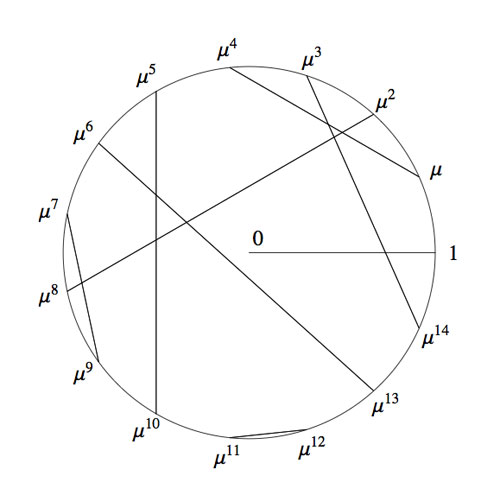Okay, we now have two identifications of the algebraic closure $\overline{\mathbb{F}_2}$ : the smaller ordinals equipped with Nim addition and Nim multiplication and the odd roots of unity with complex-multiplication and the Connes-coupling s. The question we started from asks for a general recipe to identify these two approaches.

To those of you who are convinced that finite fields (LOL, even characteristic two!) are objects far too trivial to bother thinking about : as far as I know, NOBODY knows how to do this explicitly, even restricting the ordinals to merely the natural numbers!

Please feel challenged! To get you started, I’ll show you how to place the first 15 Knights and give you a procedure (though far from explicit) to continue. Here’s the Nim-picture compatible with that above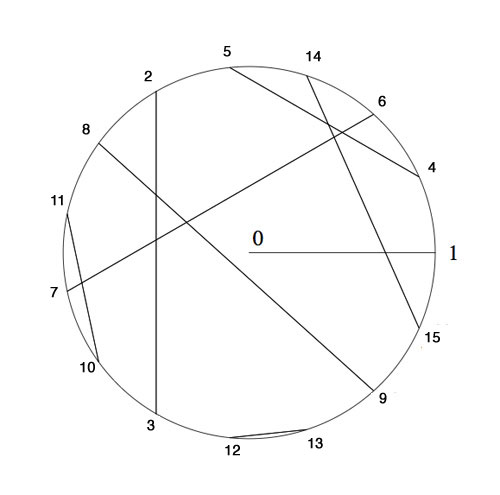To verify this, and to illustrate the general strategy, I’d better hand you the Nim-tables of the first 16 numbers. Here they are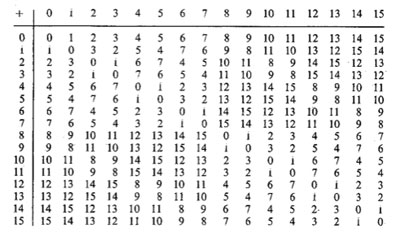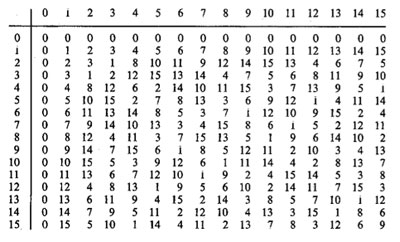It is known that the finite subfields of $~(\mathbb{N},\oplus,\otimes)$ are precisely the sets of numbers smaller than the Fermat-powers $F_n$. So, the first one is all numbers smaller than $F_1=4$ (check!). The smallest generator of the multiplicative group (of order 3) is 2, so we take this to correspond to the unit-root $e^{2 \pi i/3}$. The next subfield are all numbers smaller than $F_2 = 16$ and its multiplicative group has order 15. Now, choose the smallest integer k which generates this group, compatible with the condition that $k^{\otimes 5}=2$. Verify that this number is 4 and that this forces the identification and coupling given above.

The next finite subfield would consist of all natural numbers smaller than $F_3=256$. Hence, in this field we are looking for the smallest number k generating the multiplicative group of order 255 satisfying the extra condition that $k^{\otimes 17}=4$ which would fix an identification at that level. Then, the next level would be all numbers smaller than $F_4=65536$ and again we would like to find the smallest number generating the multiplicative group and such that the appropriate power is equal to the aforementioned k, etc. etc.

Can you give explicit (even inductive) formulae to achieve this? I guess even the problem of placing Knight 16 will give you a couple of hours to think about… (to be continued).

In ONAG, John Conway proves that the symmetric version of his recursive definition of addition and multiplcation on the surreal numbers make the class On of all Cantor’s ordinal numbers into an algebraically closed Field of characteristic two : On2 (pronounced ‘Onto’), and, in particular, he identifies a subfield
with the algebraic closure of the field of two elements. What makes all of this somewhat confusing is that Cantor had already defined a (badly behaving) addition, multiplication and exponentiation on ordinal numbers.

Over the last week I’ve been playing a bit with sage to prove a few exotic identities involving ordinal numbers. Here’s one of them ($\omega$ is the first infinite ordinal number, that is, $\omega={ 0,1,2,\ldots }$),

$~(\omega^{\omega^{13}})^{47} = \omega^{\omega^7} + 1$

answering a question in Hendrik Lenstra’s paper Nim multiplication.

However, it will take us a couple of posts before we get there. Let’s begin by trying to explain what brought this on. On september 24th 2008 there was a meeting, intended for a general public, called a la rencontre des dechiffeurs, celebrating the 50th birthday of the IHES.

One of the speakers was Alain Connes and the official title of his talk was “L’ange de la géométrie, le diable de l’algèbre et le corps à un élément” (the angel of geometry, the devil of algebra and the field with one element). Instead, he talked about a seemingly trivial problem : what is the algebraic closure of $\mathbb{F}_2$, the field with two elements? My only information about the actual content of the talk comes from the following YouTube-blurb

Alain argues that we do not have a satisfactory description of $\overline{\mathbb{F}}_2$, the algebraic closure of $\mathbb{F}_2$. Naturally, it is the union (or rather, limit) of all finite fields $\mathbb{F}_{2^n}$, but, there are too many non-canonical choices to make here.

Recall that $\mathbb{F}_{2^k}$ is a subfield of $\mathbb{F}_{2^l}$ if and only if $k$ is a divisor of $l$ and so we would have to take the direct limit over the integers with respect to the divisibility relation… Of course, we can replace this by an increasing sequence of a selection of cofinal fields such as

$\mathbb{F}_{2^{1!}} \subset \mathbb{F}_{2^{2!}} \subset \mathbb{F}_{2^{3!}} \subset \ldots$

But then, there are several such suitable sequences! Another ambiguity comes from the description of $\mathbb{F}_{2^n}$. Clearly it is of the form $\mathbb{F}_2[x]/(f(x))$ where $f(x)$ is a monic irreducible polynomial of degree $n$, but again, there are several such polynomials. An attempt to make a canonical choice of polynomial is to take the ‘first’ suitable one with respect to some natural ordering on the polynomials. This leads to the so called Conway polynomials.

Conway polynomials for the prime $2$ have only been determined up to degree 400-something, so in the increasing sequence above we would already be stuck at the sixth term $\mathbb{F}_{2^{6!}}$…

So, what Alain Connes sets as a problem is to find another, more canonical, description of $\overline{\mathbb{F}}_2$. The problem is not without real-life interest as most finite fields appearing in cryptography or coding theory are subfields of $\overline{\mathbb{F}}_2$.

(My guess is that Alain originally wanted to talk about the action of the Galois group on the roots of unity, which would be the corresponding problem over the field with one element and would explain the title of the talk, but decided against it. If anyone knows what ‘coupling-problem’ he is referring to, please drop a comment.)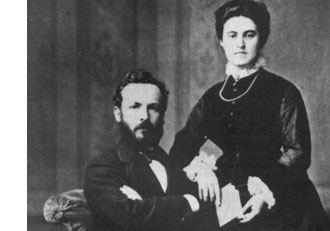Surely, Connes is aware of the fact that there exists a nice canonical recursive construction of $\overline{\mathbb{F}}_2$ due to John Conway, using Georg Cantor’s ordinal numbers.

In fact, in chapter 6 of his book On Numbers And Games, John Conway proves that the symmetric version of his recursive definition of addition and multiplcation on the surreal numbers make the class $\mathbf{On}$ of all Cantor’s ordinal numbers into an algebraically closed Field of characteristic two : $\mathbf{On}_2$ (pronounced ‘Onto’), and, in particular, he identifies a subfield

$\overline{\mathbb{F}}_2 \simeq [ \omega^{\omega^{\omega}} ]$

with the algebraic closure of $\mathbb{F}_2$. What makes all of this somewhat confusing is that Cantor had already defined a (badly behaving) addition, multiplication and exponentiation on ordinal numbers. To distinguish between the Cantor/Conway arithmetics, Conway (and later Lenstra) adopt the convention that any expression between square brackets refers to Cantor-arithmetic and un-squared ones to Conway’s. So, in the description of the algebraic closure just given $[ \omega^{\omega^{\omega}} ]$ is the ordinal defined by Cantor-exponentiation, whereas the exotic identity we started out with refers to Conway’s arithmetic on ordinal numbers.

Let’s recall briefly Cantor’s ordinal arithmetic. An ordinal number $\alpha$ is the order-type of a totally ordered set, that is, if there is an order preserving bijection between two totally ordered sets then they have the same ordinal number (or you might view $\alpha$ itself as a totally ordered set, namely the set of all strictly smaller ordinal numbers, so e.g. $0= \emptyset,1= { 0 },2={ 0,1 },\ldots$).

For two ordinals $\alpha$ and $\beta$, the addition $[\alpha + \beta ]$ is the order-type of the totally ordered set $\alpha \sqcup \beta$ (the disjoint union) ordered compatible with the total orders in $\alpha$ and $\beta$ and such that every element of $\beta$ is strictly greater than any element from $\alpha$. Observe that this definition depends on the order of the two factors. For example,$[1 + \omega] = \omega$ as there is an order preserving bijection ${ \tilde{0},0,1,2,\ldots } \rightarrow { 0,1,2,3,\ldots }$ by $\tilde{0} \mapsto 0,n \mapsto n+1$. However, $\omega \not= [\omega + 1]$ as there can be no order preserving bijection ${ 0,1,2,\ldots } \rightarrow { 0,1,2,\ldots,0_{max} }$ as the first set has no maximal element whereas the second one does. So, Cantor’s addition has the bad property that it may be that $[\alpha + \beta] \not= [\beta + \alpha]$.

The Cantor-multiplication $\alpha . \beta$ is the order-type of the product-set $\alpha \times \beta$ ordered via the last differing coordinate. Again, this product has the bad property that it may happen that $[\alpha . \beta] \not= [\beta . \alpha]$ (for example $[2 . \omega ] \not=[ \omega . 2 ]$). Finally, the exponential $\beta^{\alpha}$ is the order type of the set of all maps $f~:~\alpha \rightarrow \beta$ such that $f(a) \not=0$ for only finitely many $a \in \alpha$, and ordered via the last differing function-value.

Cantor’s arithmetic allows normal-forms for ordinal numbers. More precisely, with respect to any ordinal number $\gamma \geq 2$, every ordinal number $\alpha \geq 1$ has a unique expression as

$\alpha = [ \gamma^{\alpha_0}.\eta_0 + \gamma^{\alpha_1}.\eta_1 + \ldots + \gamma^{\alpha_m}.\eta_m]$

for some natural number $m$ and such that $\alpha \geq \alpha_0 > \alpha_1 > \ldots > \alpha_m \geq 0$ and all $1 \leq \eta_i < \gamma$. In particular, taking the special cases $\gamma = 2$ and $\gamma = \omega$, we have the following two canonical forms for any ordinal number $\alpha$

$[ 2^{\alpha_0} + 2^{\alpha_1} + \ldots + 2^{\alpha_m}] = \alpha = [ \omega^{\beta_0}.n_0 + \omega^{\beta_1}.n_1 + \ldots + \omega^{\beta_k}.n_k]$

with $m,k,n_i$ natural numbers and $\alpha \geq \alpha_0 > \alpha_1 > \ldots > \alpha_m \geq 0$ and $\alpha \geq \beta_0 > \beta_1 > \ldots > \beta_k \geq 0$. Both canonical forms will be important when we consider the (better behaved) Conway-arithmetic on $\mathbf{On}_2$, next time.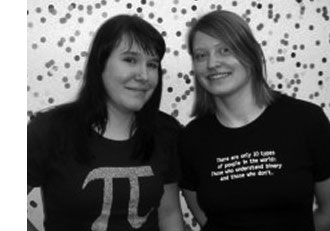Of course, excellent math-blogs exist in every language imaginable, but my linguistic limitations restrict me to the ones written in English, French, German and … Dutch. Here a few links to Dutch (or rather, Flemish) math-blogs, in order of proximity :
Stijn Symens blog, Rudy Penne’s wiskunde is sexy (math is sexy), Koen Vervloesem’s QED.

My favorite one is wiskundemeisjes (‘math-chicks’ or ‘math-girls’), written by Ionica Smeets and Jeanine Daems, two reasearchers at Leiden University. Every month they have a post called “the favorite (living) mathematician of …” in which they ask someone to nominate and introduce his/her favorite colleague mathematician. Here some examples : Roger Penrose chooses Michael Atiyah, Robbert Dijkgraaf chooses Maxim Kontsevich, Frans Oort chooses David Mumford, Gunther Cornelissen chooses Yuri I. Manin, Hendrik Lenstra chooses Bjorn Poonen, etc. the full list is here or here. This series deserves a wider audience. Perhaps Ionica and Jeanine might consider translating some of these posts?

I’m certain their English is far better than mine, so here’s a feeble attempt to translate the one post in their series they consider a complete failure (it isn’t even listed in the category). Two reasons for me to do so : it features Matilde Marcolli (one of my own favorite living mathematicians) and Matilde expresses here very clearly my own take on popular-math books/blogs.

The original post was written by Ionica and was called Weg met de ‘favoriete wiskundige van…’ :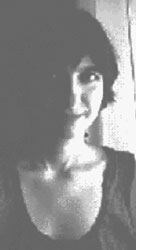“This week I did spend much of my time at the Fifth European Mathematical Congress in Amsterdam. Several mathematicians suggested I should have a chat with Matilde Marcolli, one of the plenary speakers. It seemed like a nice idea to ask her about her favorite (still living) mathematician, for our series.

Marcolli explained why she couldn’t answer this question : she has favorite mathematical ideas, but it doesn’t interest her one bit who discovered or proved them. And, there are mathematicians she likes, but that’s because she finds them interesting as human beings, independent of their mathematical achievements.

In addition, she thinks it’s a mistake to focus science too much on the persons. Scientific ideas should play the main role, not the scientists themselves. To her it is important to remember that many results are the combined effort of several people, that science doesn’t evolve around personalities and that scientific ideas are accessible to anyone.

Marcolli also dislikes the current trend in popular science writing: “I am completely unable to read popular-scientific books. As soon as they start telling anecdotes and stories, I throw away the book. I don’t care about their lives, I care about the real stuff.”

She’d love to read a popular science-book containing only ideas. She regrets that most of these books restrict to story-telling, but fail to disseminate the scientific ideas.”

Ionica then goes on to defend her own approach to science-popularization :

“… Probably, people will not know much about Galois-theory by reading about his turbulent life. Still, I can imagine people to become interested in ‘the real stuff’ after reading his biography, and, in this manner they will read some mathematics they wouldn’t have known to exist otherwise. But, Marcolli got me thinking, for it is true that almost all popular science-books focus on anecdotes rather than science itself. Is this wrong? For instance, do you want to see more mathematics here? I’m curious to hear your opinion on this.”

Even though my own approach is somewhat different, Ionica and Jeanine you’re doing an excellent job: “houden zo!”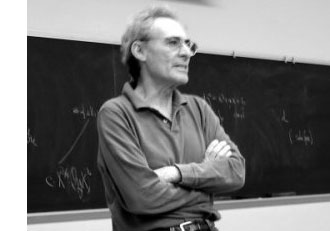In the previous posts, we have depicted the ‘arithmetic line’, that is the prime numbers, as a ‘line’ and individual primes as ‘points’.

However, sometime in the roaring 60-ties, Barry Mazur launched the crazy idea of viewing the affine spectrum of the integers, $\mathbf{spec}(\mathbb{Z})$, as a 3-dimensional manifold and prime numbers themselves as knots in this 3-manifold…

After a long silence, this idea was taken up recently by Mikhail Kapranov and Alexander Reznikov (1960-2003) in a talk at the MPI-Bonn in august 1996. Pieter Moree tells the story in his recollections about Alexander (Sacha) Reznikov in Sipping Tea with Sacha : “Sasha’s paper is closely related to his paper where the analogy of covers of three-manifolds and class field theory plays a big role (an analogy that was apparently first noticed by B. Mazur). Sasha and Mikhail Kapranov (at the time also at the institute) were both very interested in this analogy. Eventually, in August 1996, Kapranov and Reznikov both lectured on this (and I explained in about 10 minutes my contribution to Reznikov’s proof). I was pleased to learn some time ago that this lecture series even made it into the literature, see Morishita’s ‘On certain analogies between knots and primes’ J. reine angew. Math 550 (2002) 141-167.”

Here’s a part of what is now called the Kapranov-Reznikov-Mazur dictionary :What is the rationale behind this dictionary? Well, it all has to do with trying to make sense of the (algebraic) fundamental group $\pi_1^{alg}(X)$ of a general scheme $X$. Recall that for a manifold $M$ there are two different ways to define its fundamental group $\pi_1(M)$ : either as the closed loops in a given basepoint upto homotopy or as the automorphism group of the universal cover $\tilde{M}$ of $M$.

For an arbitrary scheme the first definition doesn’t make sense but we can use the second one as we have a good notion of a (finite) cover : an etale morphism $Y \rightarrow X$ of the scheme $X$. As they form an inverse system, we can take their finite automorphism groups $Aut_X(Y)$ and take their projective limit along the system and call this the algebraic fundamental group $\pi^{alg}_1(X)$.

Hendrik Lenstra has written beautiful course notes on ‘Galois theory for schemes’ on all of this starting from scratch. Besides, there are also two video-lectures available on this at the MSRI-website : Etale fundamental groups 1 by H.W. Lenstra and Etale fundamental groups 2 by F. Pop.

But, what is the connection with the ‘usual’ fundamental group in case both of them can be defined? Well, by construction the algebraic fundamental group is always a profinite group and in the case of manifolds it coincides with the profinite completion of the standard fundamental group, that is,
$\pi^{alg}_1(M) \simeq \widehat{\pi_1(M)}$ (recall that the cofinite completion is the projective limit of all finite group quotients).

Right, so all we have to do to find a topological equivalent of an algebraic scheme is to compute its algebraic fundamental group and find an existing topological space of which the profinite completion of its standard fundamental group coincides with our algebraic fundamental group. An example : a prime number $p$ (as a ‘point’ in $\mathbf{spec}(\mathbb{Z})$) is the closed subscheme $\mathbf{spec}(\mathbb{F}_p)$ corresponding to the finite field $\mathbb{F}_p = \mathbb{Z}/p\mathbb{Z}$. For any affine scheme of a field $K$, the algebraic fundamental group coincides with the absolute Galois group $Gal(\overline{K}/K)$. In the case of $\mathbb{F}_p$ we all know that this abslute Galois group is isomorphic with the profinite integers $\hat{\mathbb{Z}}$. Now, what is the first topological space coming to mind having the integers as its fundamental group? Right, the circle $S^1$. Hence, in arithmetic topology we view prime numbers as topological circles, that is, as knots in some bigger space.

But then, what is this bigger space? That is, what is the topological equivalent of $\mathbf{spec}(\mathbb{Z})$? For this we have to go back to Mazur’s original paper Notes on etale cohomology of number fields in which he gives an Artin-Verdier type duality theorem for the affine spectrum $X=\mathbf{spec}(D)$ of the ring of integers $D$ in a number field. More precisely, there is a non-degenerate pairing $H^r_{et}(X,F) \times Ext^{3-r}_X(F, \mathbb{G}_m) \rightarrow H^3_{et}(X,F) \simeq \mathbb{Q}/\mathbb{Z}$ for any constructible abelian sheaf $F$. This may not tell you much, but it is a ‘sort of’ Poincare-duality result one would have for a compact three dimensional manifold.

Ok, so in particular $\mathbf{spec}(\mathbb{Z})$ should be thought of as a 3-dimensional compact manifold, but which one? For this we have to compute the algebraic fundamental group. Fortunately, this group is trivial as there are no (non-split) etale covers of $\mathbf{spec}(\mathbb{Z})$, so the corresponding 3-manifold should be simple connected… but wenow know that this has to imply that the manifold must be $S^3$, the 3-sphere! Summarizing : in arithmetic topology, prime numbers are knots in the 3-sphere!

More generally (by the same arguments) the affine spectrum $\mathbf{spec}(D)$ of a ring of integers can be thought of as corresponding to a closed oriented 3-dimensional manifold $M$ (which is a cover of $S^3$) and a prime ideal $\mathfrak{p} \triangleleft D$ corresponds to a knot in $M$.

But then, what is an ideal $\mathfrak{a} \triangleleft D$? Well, we have unique factorization of ideals in $D$, that is, $\mathfrak{a} = \mathfrak{p}_1^{n_1} \ldots \mathfrak{p}_k^{n_k}$ and therefore $\mathfrak{a}$ corresponds to a link in $M$ of which the constituent knots are the ones corresponding to the prime ideals $\mathfrak{p}_i$.

And we can go on like this. What should be an element $w \in D$? Well, it will be an embedded surface $S \rightarrow M$, possibly with a boundary, the boundary being the link corresponding to the ideal $\mathfrak{a} = Dw$ and Seifert’s algorithm tells us how we can produce surfaces having any prescribed link as its boundary. But then, in particular, a unit $w \in D^*$ should correspond to a closed surface in $M$.

And all these analogies carry much further : for example the class group of the ring of integers $Cl(D)$ then corresponds to the torsion part $H_1(M,\mathbb{Z})_{tor}$ because principal ideals $Dw$ are trivial in the class group, just as boundaries of surfaces $\partial S$ vanish in $H_1(M,\mathbb{Z})$. Similarly, one may identify the unit group $D^*$ with $H_2(M,\mathbb{Z})$… and so on, and on, and on…

More links to papers on arithmetic topology can be found in John Baez’ week 257 or via here.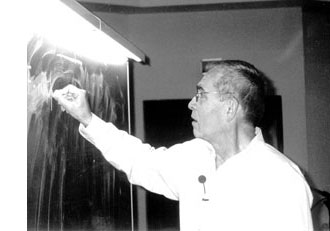Mumford’s drawing has a clear emphasis on the vertical direction. The set of all vertical lines corresponds to taking the fibers of the natural ‘structural morphism’ : $\pi~:~\mathbf{spec}(\mathbb{Z}[t]) \rightarrow \mathbf{spec}(\mathbb{Z})$ coming from the inclusion $\mathbb{Z} \subset \mathbb{Z}[t]$. That is, we consider the intersection $P \cap \mathbb{Z}$ of a prime ideal $P \subset \mathbb{Z}[t]$ with the subring of constants.

Two options arise : either $P \cap \mathbb{Z} \not= 0$, in which case the intersection is a principal prime ideal $~(p)$ for some prime number $p$ (and hence $P$ itself is bigger or equal to $p\mathbb{Z}[t]$ whence its geometric object is contained in the vertical line $\mathbb{V}((p))$, the fiber $\pi^{-1}((p))$ of the structural morphism over $~(p)$), or, the intersection $P \cap \mathbb{Z}[t] = 0$ reduces to the zero ideal (in which case the extended prime ideal $P \mathbb{Q}[x] = (q(x))$ is a principal ideal of the rational polynomial algebra $\mathbb{Q}[x]$, and hence the geometric object corresponding to $P$ is a horizontal curve in Mumford’s drawing, or is the whole arithmetic plane itself if $P=0$).

Because we know already that any ‘point’ in Mumford’s drawing corresponds to a maximal ideal of the form $\mathfrak{m}=(p,f(x))$ (see last time), we see that every point lies on precisely one of the set of all vertical coordinate axes corresponding to the prime numbers ${~\mathbb{V}((p)) = \mathbf{spec}(\mathbb{F}_p[x]) = \pi^{-1}((p))~}$. In particular, two different vertical lines do not intersect (or, in ringtheoretic lingo, the ‘vertical’ prime ideals $p\mathbb{Z}[x]$ and $q\mathbb{Z}[x]$ are comaximal for different prime numbers $p \not= q$).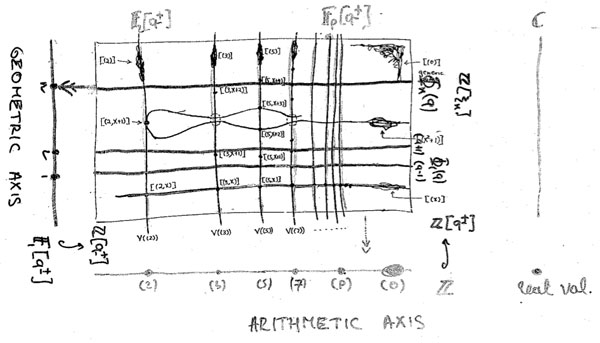That is, the structural morphism is a projection onto the “arithmetic axis” (which is $\mathbf{spec}(\mathbb{Z})$) and we get the above picture. The extra vertical line to the right of the picture is there because in arithmetic geometry it is customary to include also the archimedean valuations and hence to consider the ‘compactification’ of the arithmetic axis $\mathbf{spec}(\mathbb{Z})$ which is $\overline{\mathbf{spec}(\mathbb{Z})} = \mathbf{spec}(\mathbb{Z}) \cup { v_{\mathbb{R}} }$.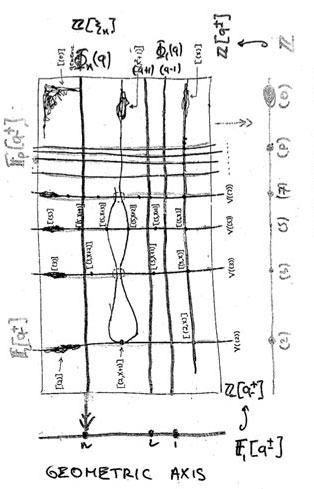Yuri I. Manin is advocating for years the point that we should take the terminology ‘arithmetic surface’ for $\mathbf{spec}(\mathbb{Z}[x])$ a lot more seriously. That is, there ought to be, apart from the projection onto the ‘z-axis’ (that is, the arithmetic axis $\mathbf{spec}(\mathbb{Z})$) also a projection onto the ‘x-axis’ which he calls the ‘geometric axis’.

But then, what are the ‘points’ of this geometric axis and what are their fibers under this second projection?

We have seen above that the vertical coordinate line over the prime number $~(p)$ coincides with $\mathbf{spec}(\mathbb{F}_p[x])$, the affine line over the finite field $\mathbb{F}_p$. But all of these different lines, for varying primes $p$, should project down onto the same geometric axis. Manin’s idea was to take therefore as the geometric axis the affine line $\mathbf{spec}(\mathbb{F}_1[x])$, over the virtual field with one element, which should be thought of as being the limit of the finite fields $\mathbb{F}_p$ when $p$ goes to one!

How many points does $\mathbf{spec}(\mathbb{F}_1[x])$ have? Over a virtual object one can postulate whatever one wants and hope for an a posteriori explanation. $\mathbb{F}_1$-gurus tell us that there should be exactly one point of size n on the affine line over $\mathbb{F}_1$, corresponding to the unique degree n field extension $\mathbb{F}_{1^n}$. However, it is difficult to explain this from the limiting perspective…

Over a genuine finite field $\mathbb{F}_p$, the number of points of thickness $n$ (that is, those for which the residue field is isomorphic to the degree n extension $\mathbb{F}_{p^n}$) is equal to the number of monic irreducible polynomials of degree n over $\mathbb{F}_p$. This number is known to be $\frac{1}{n} \sum_{d | n} \mu(\frac{n}{d}) p^d$ where $\mu(k)$ is the Moebius function. But then, the limiting number should be $\frac{1}{n} \sum_{d | n} \mu(\frac{n}{d}) = \delta_{n1}$, that is, there can only be one point of size one…

Alternatively, one might consider the zeta function counting the number $N_n$ of ideals having a quotient consisting of precisely $p^n$ elements. Then, we have for genuine finite fields $\mathbb{F}_p$ that $\zeta(\mathbb{F}_p[x]) = \sum_{n=0}^{\infty} N_n t^n = 1 + p t + p^2 t^2 + p^3 t^3 + \ldots$, whence in the limit it should become
$1+t+t^2 +t^3 + \ldots$ and there is exactly one ideal in $\mathbb{F}_1[x]$ having a quotient of cardinality n and one argues that this unique quotient should be the unique point with residue field $\mathbb{F}_{1^n}$ (though it might make more sense to view this as the unique n-fold extension of the unique size-one point $\mathbb{F}_1$ corresponding to the quotient $\mathbb{F}_1[x]/(x^n)$…)

A perhaps more convincing reasoning goes as follows. If $\overline{\mathbb{F}_p}$ is an algebraic closure of the finite field $\mathbb{F}_p$, then the points of the affine line over $\overline{\mathbb{F}_p}$ are in one-to-one correspondence with the maximal ideals of $\overline{\mathbb{F}_p}[x]$ which are all of the form $~(x-\lambda)$ for $\lambda \in \overline{\mathbb{F}_p}$. Hence, we get the points of the affine line over the basefield $\mathbb{F}_p$ as the orbits of points over the algebraic closure under the action of the Galois group $Gal(\overline{\mathbb{F}_p}/\mathbb{F}_p)$.

‘Common wisdom’ has it that one should identify the algebraic closure of the field with one element $\overline{\mathbb{F}_{1}}$ with the group of all roots of unity $\mathbb{\mu}_{\infty}$ and the corresponding Galois group $Gal(\overline{\mathbb{F}_{1}}/\mathbb{F}_1)$ as being generated by the power-maps $\lambda \rightarrow \lambda^n$ on the roots of unity. But then there is exactly one orbit of length n given by the n-th roots of unity $\mathbb{\mu}_n$, so there should be exactly one point of thickness n in $\mathbf{spec}(\mathbb{F}_1[x])$ and we should then identity the corresponding residue field as $\mathbb{F}_{1^n} = \mathbb{\mu}_n$.

Whatever convinces you, let us assume that we can identify the non-generic points of $\mathbf{spec}(\mathbb{F}_1[x])$ with the set of positive natural numbers ${ 1,2,3,\ldots }$ with $n$ denoting the unique size n point with residue field $\mathbb{F}_{1^n}$. Then, what are the fibers of the projection onto the geometric axis $\phi~:~\mathbf{spec}(\mathbb{Z}[x]) \rightarrow \mathbf{spec}(\mathbb{F}_1[x]) = { 1,2,3,\ldots }$?

These fibers should correspond to ‘horizontal’ principal prime ideals of $\mathbb{Z}[x]$. Manin proposes to consider $\phi^{-1}(n) = \mathbb{V}((\Phi_n(x)))$ where $\Phi_n(x)$ is the n-th cyclotomic polynomial. The nice thing about this proposal is that all closed points of $\mathbf{spec}(\mathbb{Z}[x])$ lie on one of these fibers!

Indeed, the residue field at such a point (corresponding to a maximal ideal $\mathfrak{m}=(p,f(x))$) is the finite field $\mathbb{F}_{p^n}$ and as all its elements are either zero or an $p^n-1$-th root of unity, it does lie on the curve determined by $\Phi_{p^n-1}(x)$.

As a consequence, the localization $\mathbb{Z}[x]_{cycl}$ of the integral polynomial ring $\mathbb{Z}[x]$ at the multiplicative system generated by all cyclotomic polynomials is a principal ideal domain (as all height two primes evaporate in the localization), and, the fiber over the generic point of $\mathbf{spec}(\mathbb{F}_1[x])$ is $\mathbf{spec}(\mathbb{Z}[x]_{cycl})$, which should be compared to the fact that the fiber of the generic point in the projection onto the arithmetic axis is $\mathbf{spec}(\mathbb{Q}[x])$ and $\mathbb{Q}[x]$ is the localization of $\mathbb{Z}[x]$ at the multiplicative system generated by all prime numbers).

Hence, both the vertical coordinate lines and the horizontal ‘lines’ contain all closed points of the arithmetic plane. Further, any such closed point $\mathfrak{m}=(p,f(x))$ lies on the intersection of a vertical line $\mathbb{V}((p))$ and a horizontal one $\mathbb{V}((\Phi_{p^n-1}(x)))$ (if $deg(f(x))=n$).
That is, these horizontal and vertical lines form a coordinate system, at least for the closed points of $\mathbf{spec}(\mathbb{Z}[x])$.

Still, there is a noticeable difference between the two sets of coordinate lines. The vertical lines do not intersect meaning that $p\mathbb{Z}[x]+q\mathbb{Z}[x]=\mathbb{Z}[x]$ for different prime numbers p and q. However, in general the principal prime ideals corresponding to the horizontal lines $~(\Phi_n(x))$ and $~(\Phi_m(x))$ are not comaximal when $n \not= m$, that is, these ‘lines’ may have points in common! This will lead to an exotic new topology on the roots of unity… (to be continued).